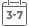# A Matrix Handbook for Statisticians

• Publish Date: 2007-11-27
• Binding: Hardcover
• Author: George A. F. Seber
• Sale
• \$222.80
• Regular price \$209.20A comprehensive, must-have handbook of matrix methods with a unique emphasis on statistical applications

This timely book, A Matrix Handbook for Statisticians, provides a comprehensive, encyclopedic treatment of matrices as they relate to both statistical concepts and methodologies. Written by an experienced authority on matrices and statistical theory, this handbook is organized by topic rather than mathematical developments and includes numerous references to both the theory behind the methods and the applications of the methods. A uniform approach is applied to each chapter, which contains four parts: a definition followed by a list of results; a short list of references to related topics in the book; one or more references to proofs; and references to applications. The use of extensive cross-referencing to topics within the book and external referencing to proofs allows for definitions to be located easily as well as interrelationships among subject areas to be recognized.

A Matrix Handbook for Statisticians addresses the need for matrix theory topics to be presented together in one book and features a collection of topics not found elsewhere under one cover. These topics include:

• Complex matrices

• A wide range of special matrices and their properties

• Special products and operators, such as the Kronecker product

• Partitioned and patterned matrices

• Matrix analysis and approximation

• Matrix optimization

• Majorization

• Random vectors and matrices

• Inequalities, such as probabilistic inequalities

Additional topics, such as rank, eigenvalues, determinants, norms, generalized inverses, linear and quadratic equations, differentiation, and Jacobians, are also included. The book assumes a fundamental knowledge of vectors and matrices, maintains a reasonable level of abstraction when appropriate, and provides a comprehensive compendium of linear algebra results with use or potential use in statistics. A Matrix Handbook for Statisticians is an essential, one-of-a-kind book for graduate-level courses in advanced statistical studies including linear and nonlinear models, multivariate analysis, and statistical computing. It also serves as an excellent self-study guide for statistical researchers.

MORE FROM THIS COLLECTION